# 2. 子空间

• 包含0向量
• 若x是V中的一个向量，那么x与一个变量的乘积$$nx$$也在线性子空间V中——数乘封闭性
• 若a，b是V中的两个向量，那么a+b也在线性子空间V中——加法封闭性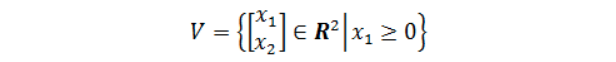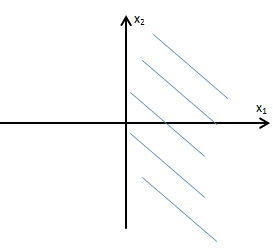• 由于a1+a2 ≥ 0，所以满足加法封闭性。
• 检验数乘封闭性: 当标量为负数时，得到的结果$$-x$$，超越了第一、四象限，不在这个子空间V中，不满足数乘封闭性
• 因此，V不是$$R^2$$的子空间

# 3. 零空间

A是$$m\times n$$矩阵，x是列向量，如果存在向量集合N，满足

$N=\{x \in R^n | Ax =0 \}$

## 3.1. 零空间与"Ax=0"1. 加法封闭性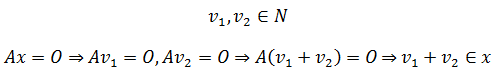1. 数乘封闭性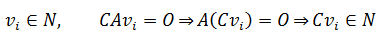## 3.2. 找出零空间

### 3.2.1. 例子1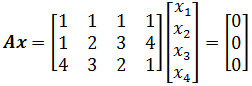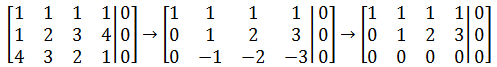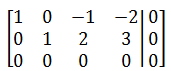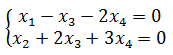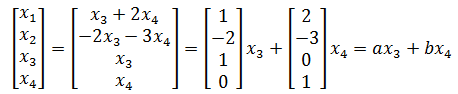x3，x4可以是任意实数，a，b是线性无关的，所以A的零空间就是a和b张成的空间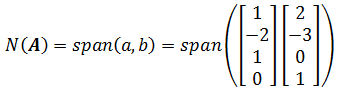### 3.2.2. 例子2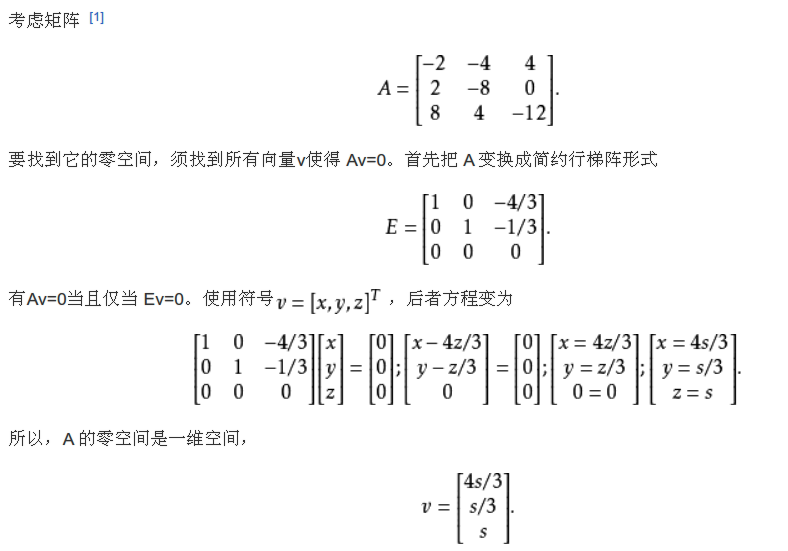## 3.3. 零空间与线性无关

### 3.3.1. 线性相关与线性无关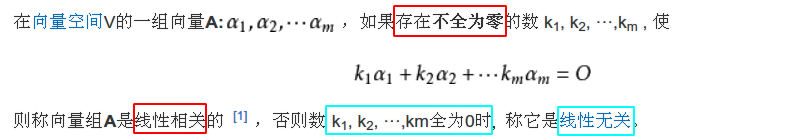### 3.3.2. 线性无关矩阵的零空间只包含零向量

$$A_{m\times n}$$由n个列向量组成，那么Ax即表示了对n个列向量的线性组合，即: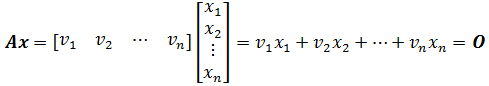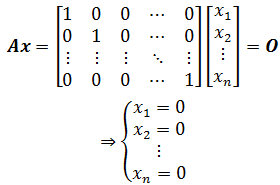• 当A是满秩方阵或列满秩的长方矩阵时，A的零空间只有零向量（仅仅是行满秩时就不一定了）
• 当一个矩阵的零空间只有零向量时，说明该矩阵的列向量线性无关

# 4. 列空间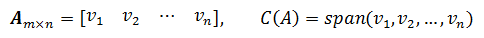## 4.1. 列空间与"Ax=b"

$Ax=b$

• 如果b在矩阵A的列空间中(即b可以使用Ax来表示(x不全为0))，此时Ax = b有解
• b不在A的列空间中，Ax = b无解